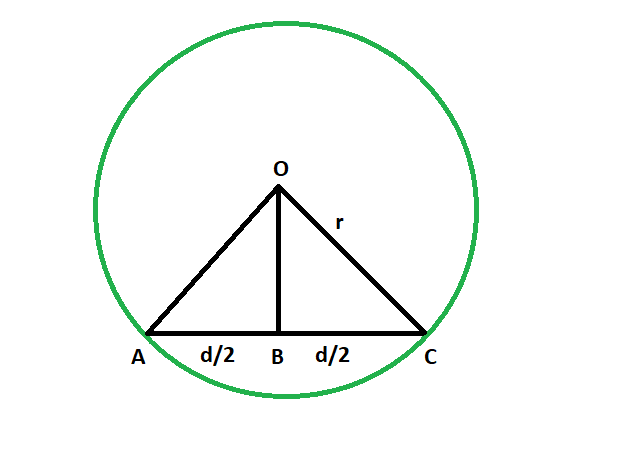# Shortest distance from the centre of a circle to a chord

Given a circle which has a chord inside it. The length of the chord and the radius of the circle are given. The task is to find the shortest distance from the chord to the centre.

Examples:

```Input: r = 4, d = 3
Output: 3.7081

Input: r = 9.8, d = 7.3
Output: 9.09492
```Approach:

• We know that the line segment that is dropped from the centre on the chord bisects the chord. The line is the perpendicular bisector of the chord, and we know the perpendicular distance is the shortest distance, so our task is to find the length of this perpendicular bisector.
• let radius of the circle = r
• length of the chord = d
• so, in triangle OBC,

from pythagorus theorem,
OB^2 + (d/2)^2 = r^2
so, OB = √(r^2 – d^2/4)

• So,• Below is the implementation of the above approach:

## C++

 `// C++ program to find ` `// the shortest distance from ` `// chord to the centre of circle ` `#include ` `using` `namespace` `std; ` ` `  `// Function to find the shortest distance ` `void` `shortdis(``double` `r, ``double` `d) ` `{ ` `    ``cout << ``"The shortest distance "` `         ``<< ``"from the chord to centre "` `         ``<< ``sqrt``((r * r) - ((d * d) / 4)) ` `         ``<< endl; ` `} ` ` `  `// Driver code ` `int` `main() ` `{ ` `    ``double` `r = 4, d = 3; ` `    ``shortdis(r, d); ` `    ``return` `0; ` `} `

## Java

 `// Java program to find  ` `// the shortest distance from  ` `// chord to the centre of circle  ` `class` `GFG ` `{ ` `     `  `// Function to find the shortest distance  ` `static` `void` `shortdis(``double` `r, ``double` `d)  ` `{  ` `    ``System.out.println(``"The shortest distance "` `        ``+ ``"from the chord to centre "` `        ``+ (Math.sqrt((r * r) - ((d * d) / ``4``))));  ` `}  ` ` `  `// Driver code  ` `public` `static` `void` `main(String[] args) ` `{ ` `    ``double` `r = ``4``, d = ``3``;  ` `    ``shortdis(r, d);  ` `} ` `} ` ` `  `/* This code contributed by PrinciRaj1992 */`

## Python3

 `# Python program to find  ` `# the shortest distance from  ` `# chord to the centre of circle  ` ` `  `# Function to find the shortest distance  ` `def` `shortdis(r, d):  ` `    ``print``(``"The shortest distance "``,end``=``""); ` `    ``print``(``"from the chord to centre "``,end``=``""); ` `    ``print``(((r ``*` `r) ``-` `((d ``*` `d) ``/` `4``))``*``*``(``1``/``2``)); ` ` `  `# Driver code  ` `r ``=` `4``; ` `d ``=` `3``;  ` `shortdis(r, d);  ` ` `  `# This code has been contributed by 29AjayKumar `

## C#

 `// C# program to find  ` `// the shortest distance from  ` `// chord to the centre of circle  ` `using` `System; ` ` `  `class` `GFG  ` `{  ` `     `  `    ``// Function to find the shortest distance  ` `    ``static` `void` `shortdis(``double` `r, ``double` `d)  ` `    ``{  ` `        ``Console.WriteLine(``"The shortest distance "` `            ``+ ``"from the chord to centre "` `            ``+ (Math.Sqrt((r * r) - ((d * d) / 4))));  ` `    ``}  ` `     `  `    ``// Driver code  ` `    ``public` `static` `void` `Main()  ` `    ``{  ` `        ``double` `r = 4, d = 3;  ` `        ``shortdis(r, d);  ` `    ``}  ` `}  ` ` `  `// This code is contributed by AnkitRai01 `

## PHP

 ` `

Output:

```The leshortest distance from the chord to centre 3.7081
```

My Personal Notes arrow_drop_upCheck out this Author's contributed articles.

If you like GeeksforGeeks and would like to contribute, you can also write an article using contribute.geeksforgeeks.org or mail your article to contribute@geeksforgeeks.org. See your article appearing on the GeeksforGeeks main page and help other Geeks.

Please Improve this article if you find anything incorrect by clicking on the "Improve Article" button below.

Article Tags :
Practice Tags :

Be the First to upvote.

Please write to us at contribute@geeksforgeeks.org to report any issue with the above content.